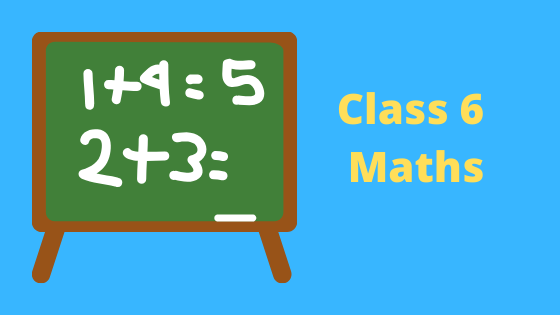# Class 6 Maths Study Material | NCERT solutions | Worksheets

•• Welcome to our class 6 Maths page. In this page you can find class 6 Maths ncert solutions , notes, assignments, worksheets etc. You can share the link to free Class 6 NCERT Maths solutions with your classmates as all study material is free of cost.

1. First read and understand the notes.
2. Try to go through the solved examples of the chapter you are reading
3. Now it is the time to solve unsolved assignments and worksheets. You will find plenty of them here or on the respective chapter page.

## Frequently asked Questions on CBSE Class 6 Maths

### How many chapters are there in Class 6 Maths?

There are total 12 chapters in Class 6 Maths as per the rationalized Syllabus. All the chapters are required for assessment

### What is the best books for Class 6 Maths?

Couple of book
(a)NCERT Class 6 Maths textbook
(b)NCERT Class 6 Exemplar
(c)RD Sharma or RS agarwal

### Which is the easiest chapter of class 6 Maths NCERT Text book?

Knowing your numbers and Whole number are the easiest chapter

### Is Class 6 Maths NCERT Text book Sufficient for CBSE Exams?

It is sufficient if you go through all the questions in the NCERT text book thoroughly and practice them all.However to enhance your maths skill , you can go for NCERT exemplar and other books

### what is the best way to learn the concept in Class 6 Maths?

First go through all the Notes and solved examples and Then do the exercises. Practice Lot of questions. The simple Mantra is Practice .Practice and Practice

### what is the deleted syllabus in Class 6 Maths?

Chapter 1: Knowing Our Numbers
Estimation,Using Brackets,Expanding brackets,Roman Numerals
Chapter 2: Whole Numbers
Properties of whole numbers,Patterns in whole numbers
Chapter 3: Playing with Numbers
Some more divisibility rules
Chapter 4: Basic Geometrical Ideas
Chapter 5: Understanding Elementary Shapes
Three dimensional shapes
Chapter 8: Decimals
Tenths,Hundredths
Chapter 9: Data Handling
Drawing a pictograph,A bar graph
Chapter 11: Algebra
Use of variables in common rules,Expressions with variables,Using expressions practically,What is an equation?,Solution of an equation
Chapter 13: Symmetry
Whole Chapter Deleted
Chapter 14: Practical Geometry
Whole Chapter Deleted### Practice Question

Question 1 What is $\frac {1}{2} + \frac {3}{4}$ ?
A)$\frac {5}{4}$
B)$\frac {1}{4}$
C)$1$
D)$\frac {4}{5}$
Question 2 Pinhole camera produces an ?
A)An erect and small image
B)an Inverted and small image
C)An inverted and enlarged image
D)None of the above